# How do succinct data types work in libcds?

Libcds is a library which implements low-level succinct data structures to provide the building blocks of most compressed/succinct solutions. Libcds is written in C++.

There are 4 kind of succinct data structures in libcds which are based on bitmaps:

• DArray
• RG
• RRR
• SDArray

## What is common in these structures?

Each structure can be built from a BitString. BitString is the data container of libcds which consists of the following:

```size_t length;
size_t uintLength;
uint * data;```

“length” is the count of bits (of the whole data).
“uintLength” is the count of uints at the memory where the “data” pointer points.
“data” is pointer to uints which hold the data.

size_t is at least 32 bit on 32 bit systems and at least 64 bit on 64 bit machines (because it’s the type of the size parameter of malloc). So you can hold a maximum of 64^2 bits in this structure on a 64 bit system.

## How does RG work?

#### Basics

RG uses a superblock table where each element tells how many ones present till it’s corresponding data group. When you create this structure you have to define a factor which tells how many data blocks must be grouped together.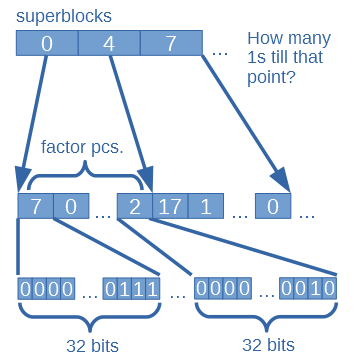#### Building the RG structure

When you build an RG structure you have to define the bitstring and a factor.

`BitSequenceRG::BitSequenceRG(const BitString & bs, uint _factor);`

bs is the source data
_factor means that how many uints of bs will make a superblock

This class holds the following variables:

```size_t n = bitcount of bs
size_t integers = number of uints in bs
size_t factor = _factor
size_t s = 32 * factor
uint *data;
uint *Rs;```

s is the number of bits in a superblock.
Rs is the array of superblocks.

The constructor function creates an uint array named “data” with length “integers”. The uints from bs copied here. The remaining space is filled with zeros.

Then it builds the array of superblocks by calling “void BitSequenceRG::BuildRank()”.

The superblock array (Rs) is filled up in this way:

1. The first element is 0 because rank is obviously 0 before the first bit of data.
2. The further elements are calculated as: the previous element + the number of ones in the previous block of “data” (with block size of “s” bits).

#### How does rank work on RG?

`size_t BitSequenceRG::rank1(const size_t i1);`

i1 is the index where we want the rank.

First it gets the element with index i1/s from the superblock array (Rs).
This will tell the number of ones till the block’s start (where the index maps).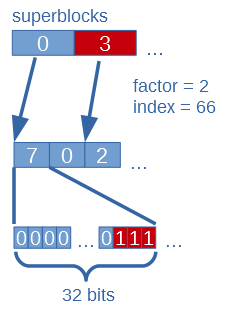Second it iterates through all uints in data from block’s start to just before the uint comes where the index maps. It counts ones in these uints and sums them. The uint where the index maps has to be masked before it can count the ones in it (because the remaining part must be dismissed). Than number of ones in it are counted and summed with the previous result.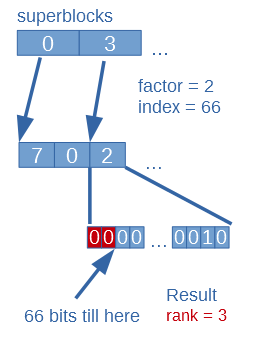This result is the rank.

#### How does select work on RG?

`size_t BitSequenceRG::select1(const size_t x1);`

We are looking for the index of the x1-th one in data.
x1 parameter is stored as x variable in this function.

First it does a binary search in the superblocks array (Rg) until it finds the greatest element which is less than x. This means that where this superblock maps (start of uint block in “data”) there are fewer ones than x.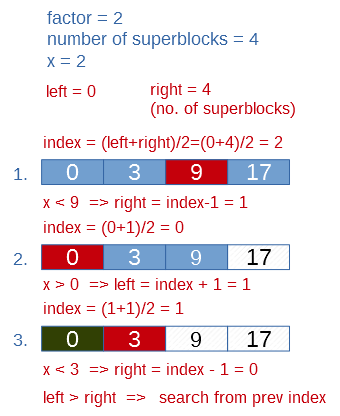Second it subtracts the found superblock element from x. So x will equal to the remaining ones which need to be counted from this block.

( in the example the found element is 0, so  x won’t change )

Third it iterates the uints from this block and subtracts the number of ones in them from x. This goes until a block which has more ones than the current x. It means that the select result will be in this uint block. This block’s value is saved and future work is done on it.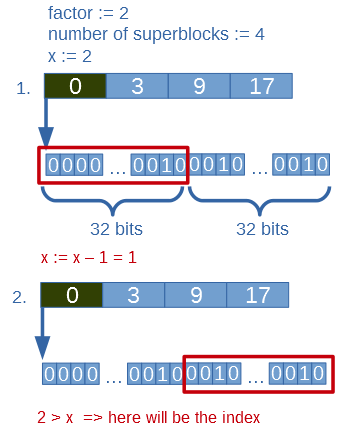Fourth it iterating through 8 bit parts of this uint (by shifting and substracing number of ones of these parts) until a 8 bit part has more or equal ones than the current x.Fifth it iterates through this 8 bit part (bit by bit) by shifting and subtracts the current bit value by masking from x.

It returns the bit index where the last one has been found.

Note:
RG uses popcount and popcount8 function where it counts the number of ones in a 8/32 bit variable. These functions use array “const unsigned char __popcount_tab[]”, which is a lookup table with 256 elements.

For a 8 bit value it gets the number of ones in step (be using the value as the index of the table). So this table contains the number of ones of every possible 8 bit value.

For a 32 bit value it gets the number of ones by making 4 x 8 bits from it (by masking) and summing their value in the lookup table.

#### Time and space requirement

n – data length in bits
factor – how many blocks to group together (for superblocks)

Building the structure:
time(n, factor) = O(n)
space(n, factor) = O(n/factor)*

* It’s O(n) in the current implementation because it makes a copy of the original data, but it can be easily fixed.

Rank:
time(n, factor) = O(factor)

Select:
time(n, factor) = O(log(n/factor)) + O(factor)

#### Problems and inefficiencies in RG

• Every array elements are copied one by one. Zeroing is also done one by one. A standard library call should be used instead!
• There are two elements which have the same meaning: constant “W” in cds-utils namespace and “b” a class variable in BitSequenceRG. They are set to 32 (the bit-length of uint). It is a bad practice to use two variables for the same purpose (maybe you forget to change both). In my notes I changed them to constant 32.
• uint is used everywhere in the code. Because uint is not a standard C++ data type the code will not work consistently (or at all) across different platforms. On my 64 bit machine with Linux and GCC 4.8.2/Clang 3.3 uint is defined as unsigned int and it is a 32 bit data type.
• On 64 bit platforms a 64 bit storage type (like uint64_t) should be used for performance
• popcount functions are great however on modern processors it could be optimized to one opcode by using assembly.

## 2 thoughts on “How do succinct data types work in libcds?”

1.András Majdán Post author

Kind of.. There are multiple structures in libcds, one of it is RG. This post describes how RG works.

This site uses Akismet to reduce spam. Learn how your comment data is processed.